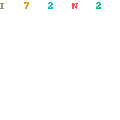# Fuzzy Operator Theory in Mathematical AnalysisFuzzy Operator Theory in Mathematical Analysis by Yeol Je Cho
English | PDF,EPUB | 2018 | 411 Pages | ISBN : 3319934996 | 26.7 MB

This self-contained monograph presents an overview of fuzzy operator theory in mathematical analysis. Concepts, principles, methods, techniques, and applications of fuzzy operator theory are unified in this book to provide an introduction to graduate students and researchers in mathematics, applied sciences, physics, engineering, optimization, and operations research.

New approaches to fuzzy operator theory and fixed point theory with applications to fuzzy metric spaces, fuzzy normed spaces, partially ordered fuzzy metric spaces, fuzzy normed algebras, and non-Archimedean fuzzy metric spaces are presented.
Surveys are provided on: Basic theory of fuzzy metric and normed spaces and its topology, fuzzy normed and Banach spaces, linear operators, fundamental theorems (open mapping and closed graph), applications of contractions and fixed point theory, approximation theory and best proximity theory, fuzzy metric type space, topology and applications.